Your Dashboardusername@email.com

# Numbers in Their Prime

#### Objective

In this lesson we will review prime and composite numbers. We’ll also review some handy rules that make division faster and easier.

#### Previously Covered

• So far, we’ve covered the various types of numbers (real numbers, rational numbers, irrational numbers, whole numbers, and integers), as well as how they relate to each other using the common device of a number line.
• We’ve also discussed the various properties of addition and multiplication.
All whole numbers are categorized as either prime or composite, with the exceptions of zero and one, which are special cases. Let’s take a look at the difference between prime and composite numbers.  Prime numbers have exactly two factors, one and the number itself.  If you know your divisibility rules (covered in the next section), then determining the prime numbers from 1–100 is a relatively easy task. Here are the prime numbers up to 25:

2, 3, 5, 7, 11, 13, 17, 19, 23

There is no pattern for finding all of the prime numbers that exist, although mathematicians have found prime numbers with almost eight million digits. This is one of the remaining big math mysteries out there for mathematicians.

Composite numbers have more than two factors but not an infinite number of factors. All even numbers (except the number two) are composite, since they can all be divided by two.

Zero is neither prime nor composite. Since any number times zero equals zero, there are an infinite number of factors for a product of zero. A composite number must have a finite number of factors.

One is also neither prime nor composite. The only way to get a product of one is by multiplying 1 x 1. But duplicate factors are only counted once, so one only has one factor. (A prime number has exactly two factors, so one can’t be prime.)

A typical test question would have you identify which number from a list is prime (or composite or neither).

Try this one:

#### Question

Which of the following is a prime number?

1. 33
2. 45
3. 41
4. 51

The correct answer is C. Forty-one is a prime number; the other numbers are composite. Be careful with 51—it comes from 3 x 17.

### Cool Rules

Divisibility rules are tools to help you do less division. The divisibility rules can help you determine if a number is prime or composite as well as help you break composite numbers into their prime factors, the topic we will cover next.

Divisibility rules are shortcuts to tell you if one number is divisible by another number. The rules cover division by numbers 2–10 and allow you to check numbers up through 100. Click on the numbers in the chart.

Divisibility Rules
2 3 4
5 6 7
8 9 10

#### Question

Which of the following numbers is not divisible by 3?

1. 4,873
2. 7,521
3. 12,642
4. 25,308

The correct answer is A. The fast way to do this problem is to use the divisibility rule; add the digits of each number looking for the one that is not a multiple of three. The slow way would be to do each division problem—even on a calculator, this method will take longer!

### Prime Factorization: T’s and Tree

This is another topic you might not have thought about since middle school. The Fundamental Theorem of Arithmetic states that every composite number can be broken down into a unique product of prime numbers.

Prime factorization is the process that finds the prime-number products of a given composite number. Prime factorization is useful in its own right, but many of the applications for it involve fractions, which we’ll cover down the road.

You may be able to find a number’s prime factorization in your head. For example, 12 is 2 x 2 x 3 or 22 x 3. (Prime factorizations are often represented with exponents.)

If you can’t find the prime factorization mentally, try either a Factor-T or a Factor Tree.

#### Factor-T

In a Factor-T, you will have two columns: the left column with the prime numbers and the right column with progressively smaller quotients from division.

Step 1: Determine a prime number that divides into your given number. Write that number in the left column.

Step 2: Write the quotient of your number divided by the prime number in the right hand column.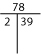Step 3: Now look for a prime number that divides into the quotient, 39 in this case. Continue steps 1 and 2 until you have a one in the right-hand column. The numbers on the left are your prime factorization!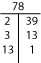The prime factorization of 78 is 2 x 3 x 13. No other number has this prime factorization.

Here is another example for the prime factorization of 200.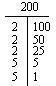The prime factorization of 200 is 23 x 52.

#### Factor Tree

With a factor tree, you are always breaking a number into the product of two numbers until the numbers are prime. There are many tactics for getting started at the top of the tree, which means that the trees will not necessarily look the same when made by different people. Use whatever works best for you to figure out any two numbers that will multiply together to make the number you need.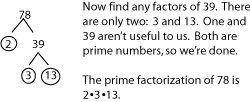Here is another example for the prime factorization of 200.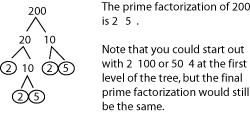### Review

• Prime numbers have exactly two factors, one and itself.
• Composite numbers have more than two factors but not an infinite number.
• Zero and one are neither prime nor composite.
• Divisibility rules are shortcuts that can assist you in determining factors of a number.
• Every composite number can be written as a unique product of prime numbers, which is called a number’s prime factorization.
• Two handy methods of finding prime factorizations are factor-T’s and factor trees.